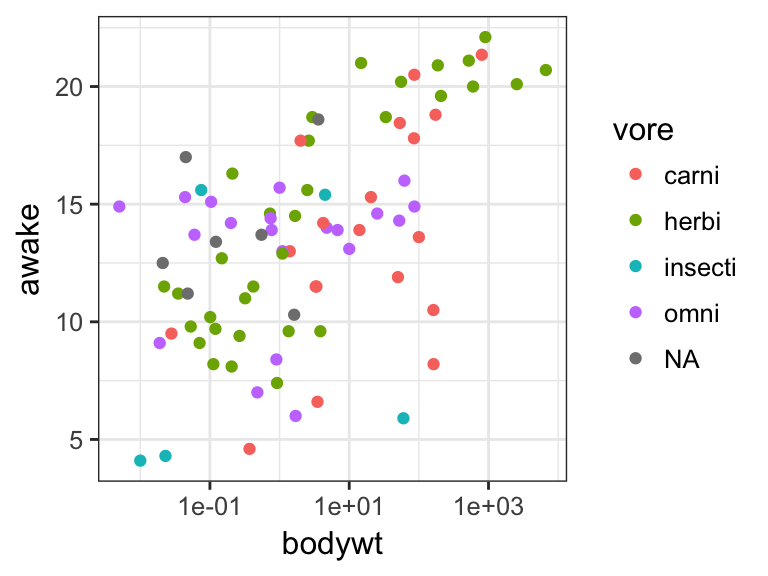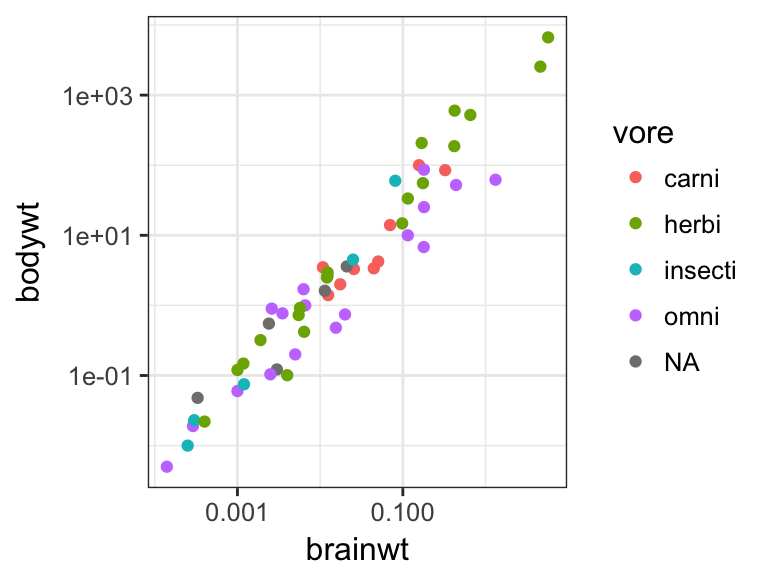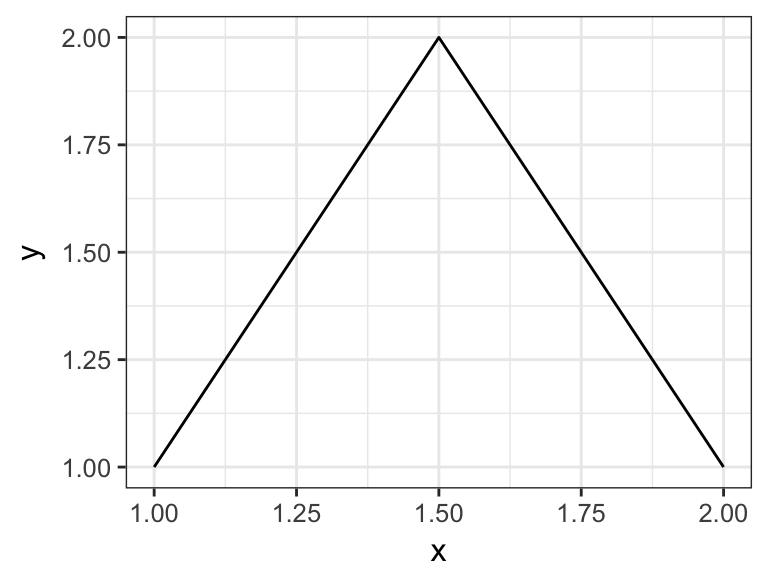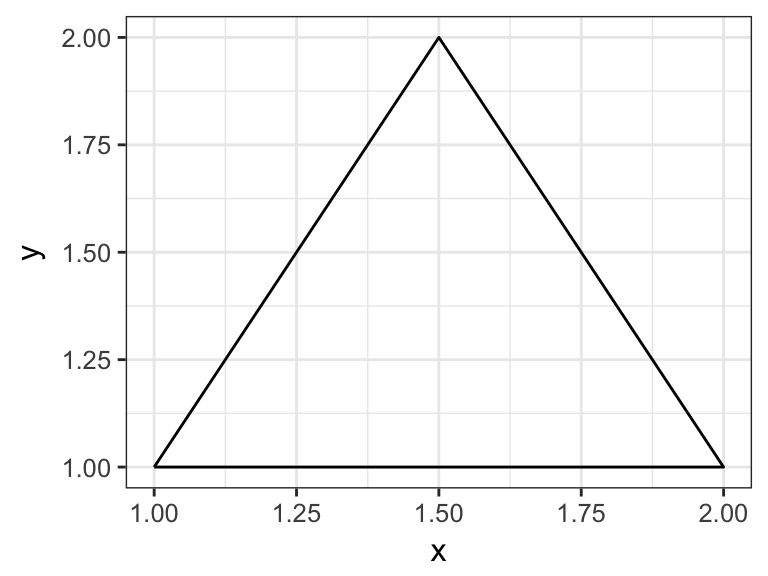## Lab Worksheet 2

We will be working with the msleep data set that is provided with ggplot2. The data set contains information about the sleep habits of 83 mammals. Enter ?msleep on the R command line to learn more about the dataset.

Problem 1: Make the following plots: (i) a plot of total time awake vs. body weight, colored by “vore” (carnivore, herbivore, etc.); (ii) a plot of body weight vs. brain weight, colored by “vore”. When you plot body weight and/or brain weight, consider whether a linear scale or a logarithmic scale seems more appropriate, and explain your reasoning in 1-2 sentences. HINT: Use the functions scale_x_log10() and scale_y_log10() to change the scales.

ggplot(msleep, aes(x=bodywt, y=awake, color=vore)) + geom_point() + scale_x_log10()ggplot(msleep, aes(x=brainwt, y=bodywt, color=vore)) + geom_point() + scale_x_log10() + scale_y_log10()
## Warning: Removed 27 rows containing missing values (geom_point).Log scales are more appropriate for both body weight and brain weight because there are a few species that have much larger values than most other species. If we were to use a linear scale, these outlying species would require axis ranges that are too wide to resolve any details for the majority of the species.

Problem 2: Explain the difference between geom_line() and geom_path(). Make up a simple data set (5-10 data points), plot it twice, once using geom_line() and once using geom_path(), and explain why each plot looks the way it does.

geom_line() connects data points in the order from smallest to largest x value. geom_path(), by contrast, connects data points in the order in which they appear in the data frame. The following data set produces an open triangle with geom_line() and a closed triangle with geom_path().

d <- data.frame(x=c(1, 2, 1.5, 1), y=c(1, 1, 2, 1))
ggplot(d, aes(x=x, y=y)) + geom_line()ggplot(d, aes(x=x, y=y)) + geom_path()